# Spencer Woodworth - Tangent Space Normal Mapping of Cisterns From Stereo Images

## Data Collection

First we captured stereo data using the GoPro Hero 3D system.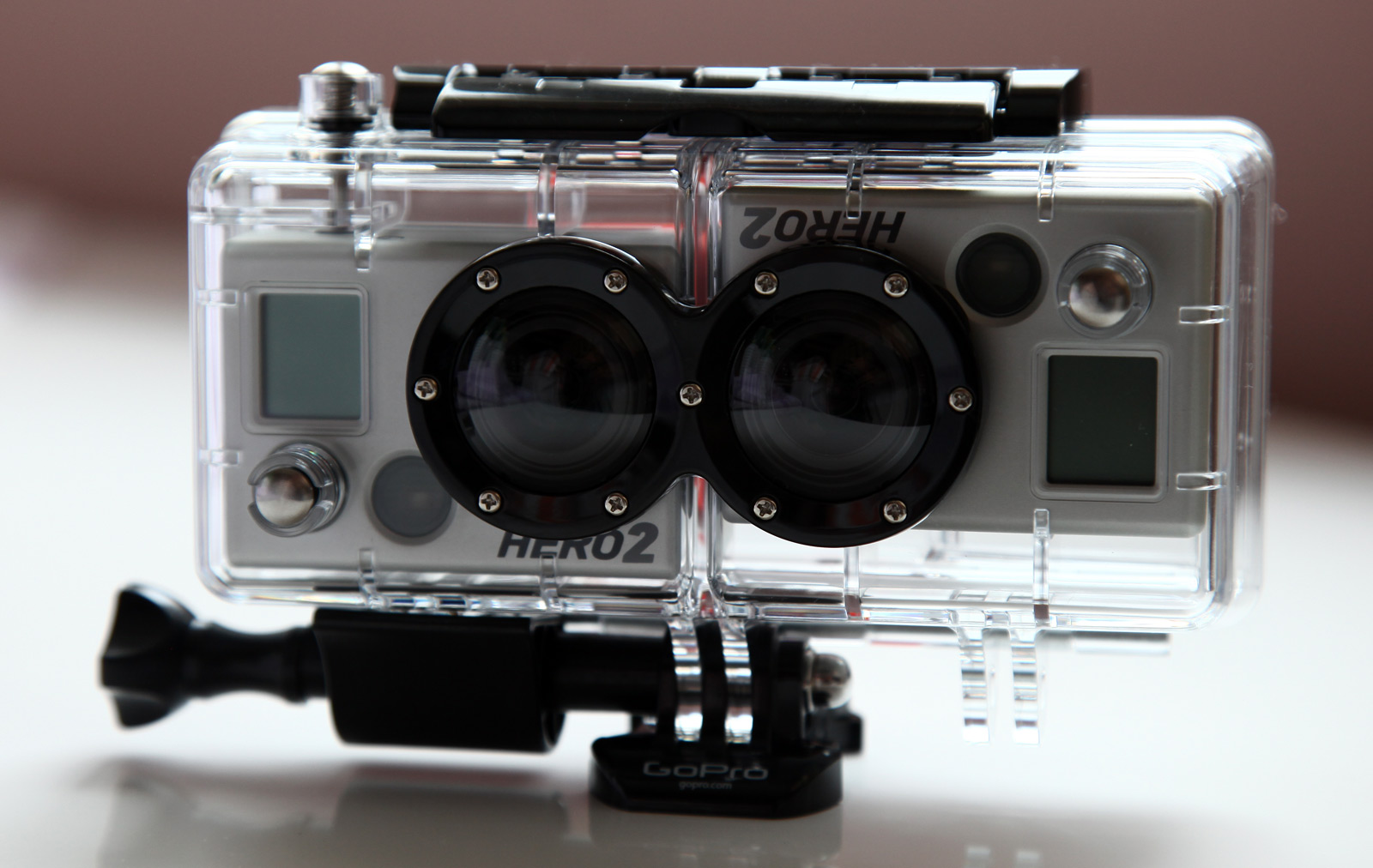Some examples of left and right images are below.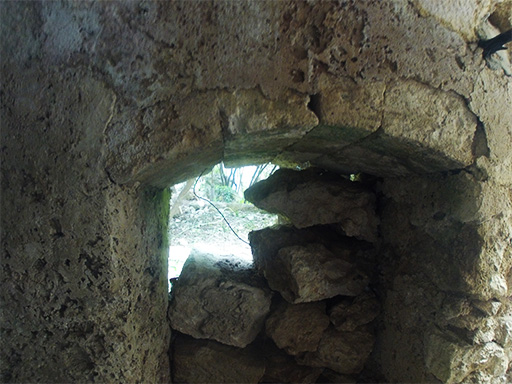Left GoPro Image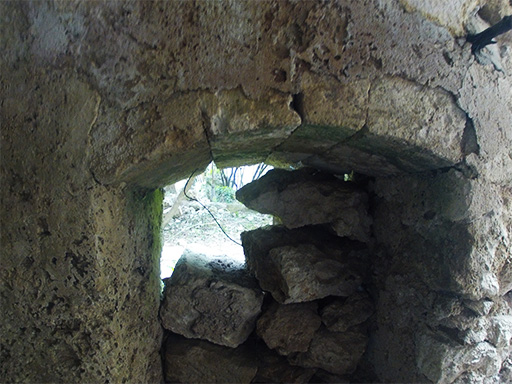Right GoPro Image

## Disparity Calculation

Then we built a disparity map using MatLab. The results of the left and right image shown above: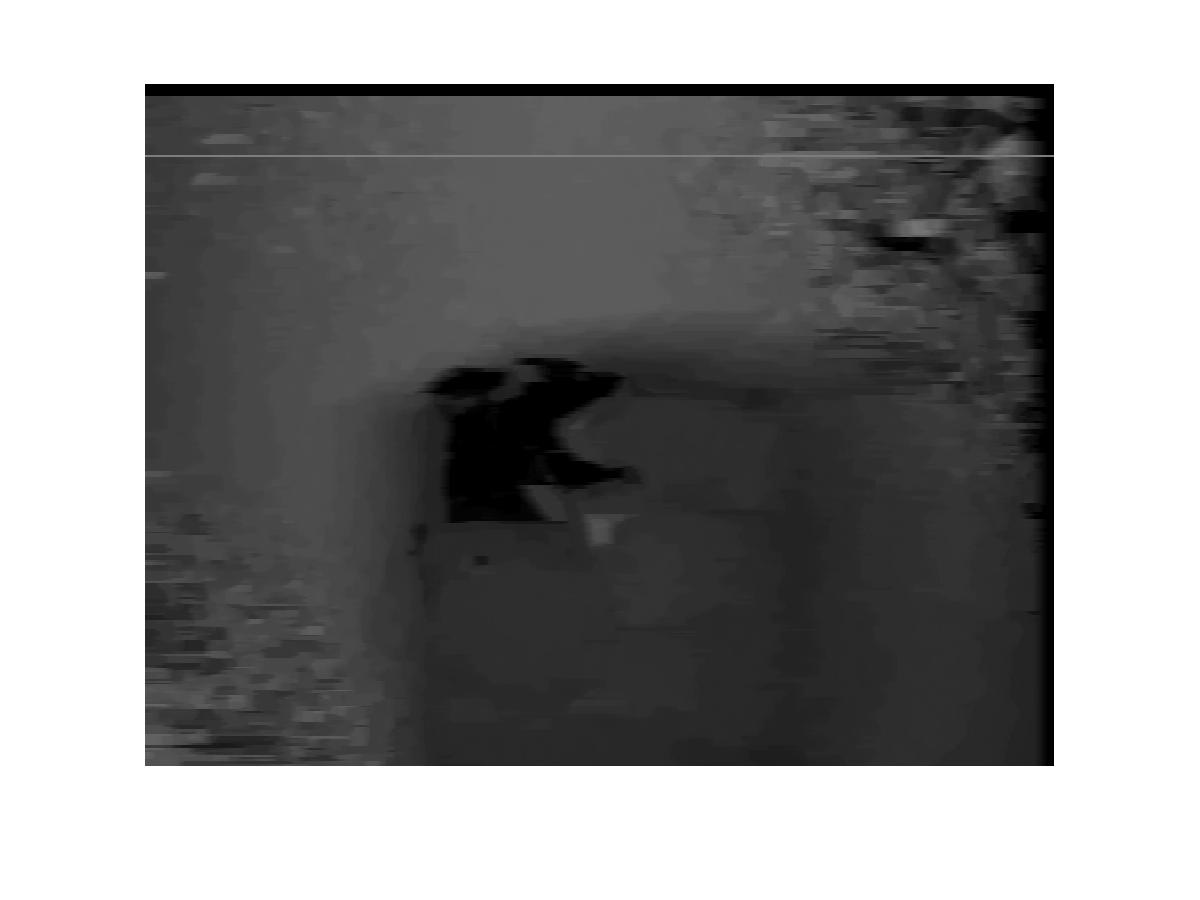## Normal Map Creation

Next we build a normal map from the disparity image by comparing neighboring pixels and shifting the normal accordingly.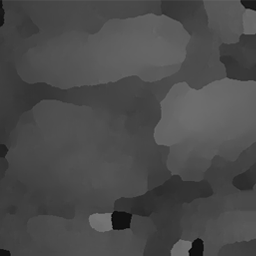Disparity Map Output From MatLab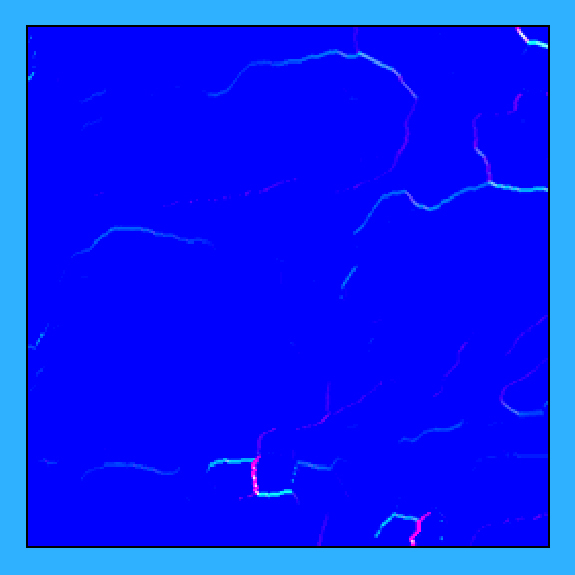Normal Map Displayed As RGB Values

I then recalculate phong shading using using these new normals.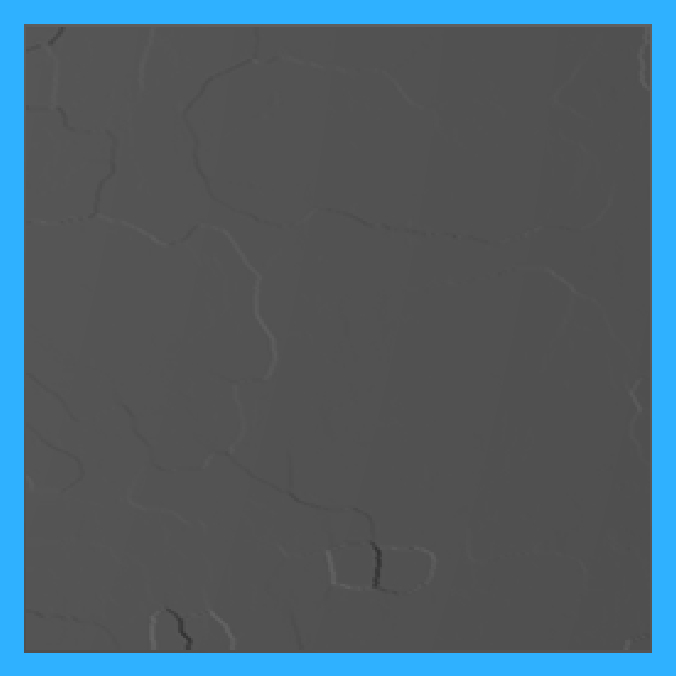Phong Shading Using Normal Map

Then the original texture image is added.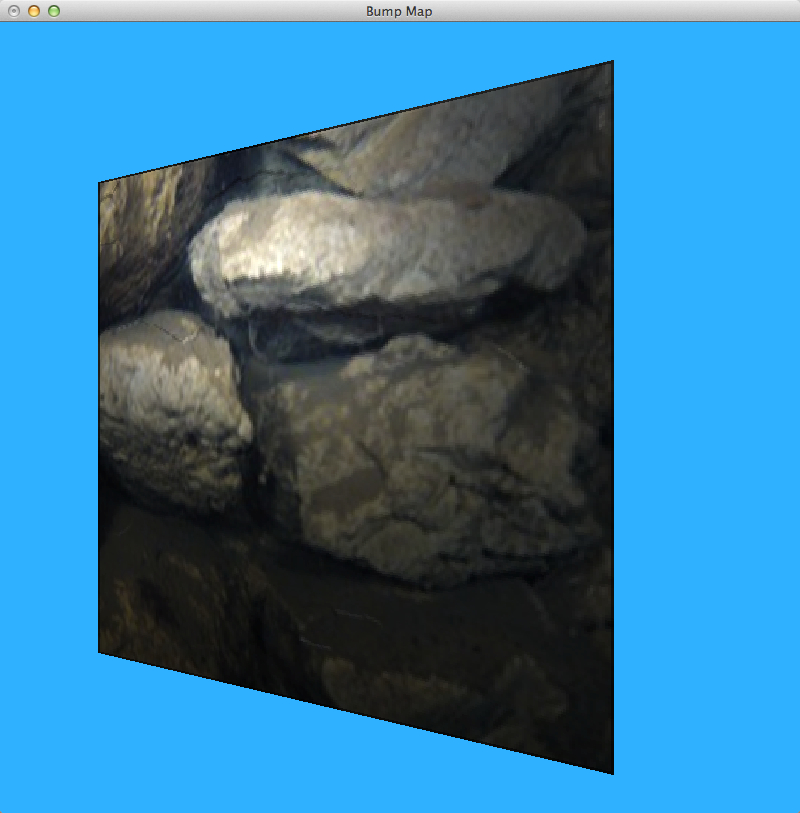## Normals Transformed Into Tangent Space

Finally, I transform the normals into tangent space. For this demo I have hardcoded the TBN matrices. I have removed the texture to make the results more noticeable.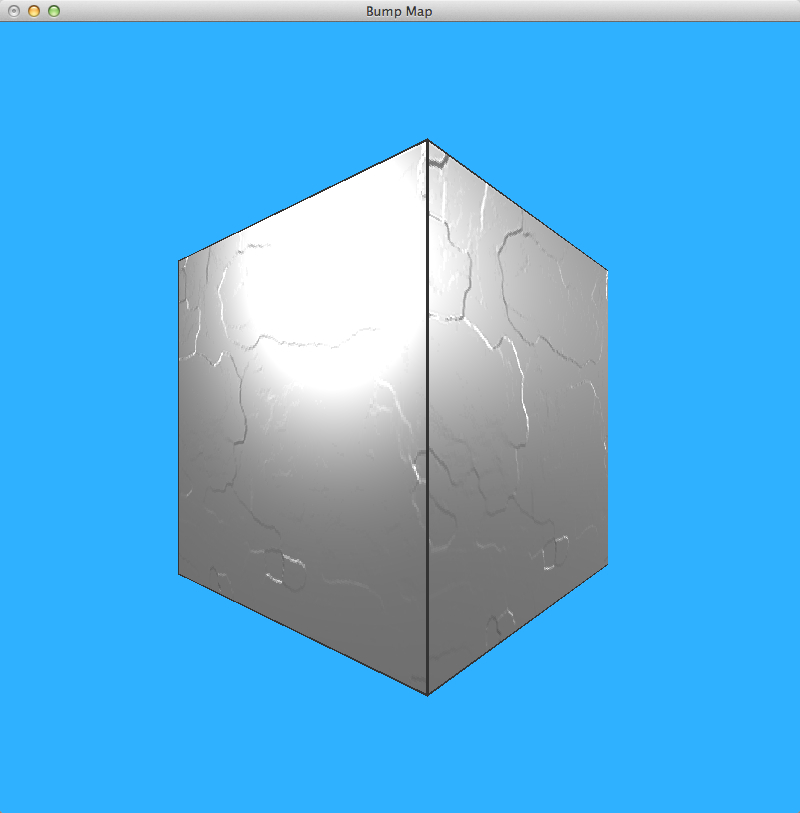## Future Work

• Change normal map calculation to use cross products rather than neighbor differences
• Calculate a separate TBN matrix for each vertex in a cistern model
• Improved disparity map calculations
• Improved stereo image data collection techniques